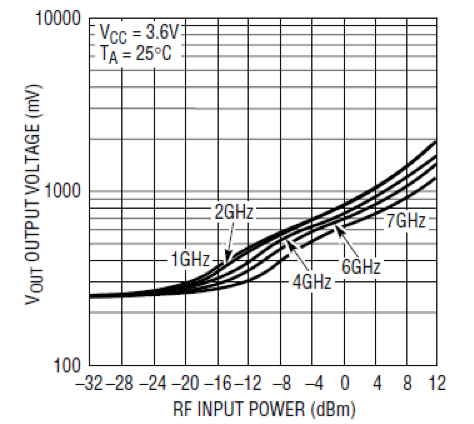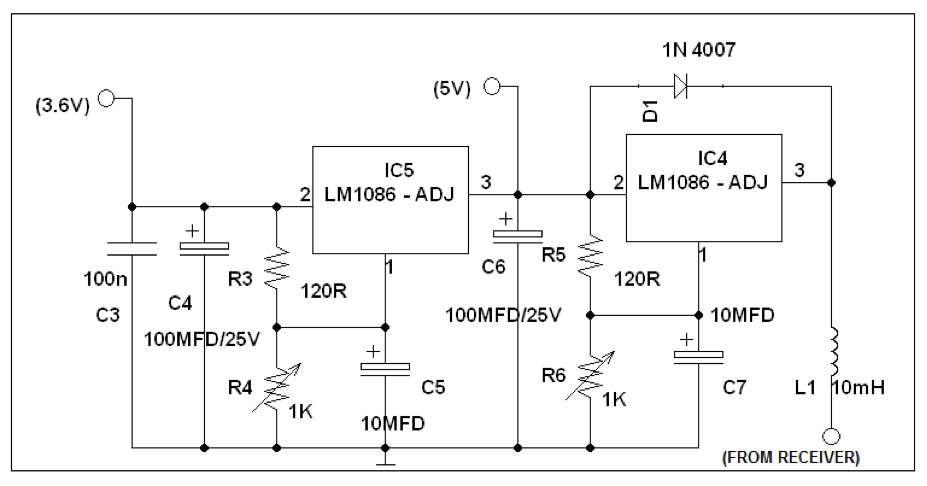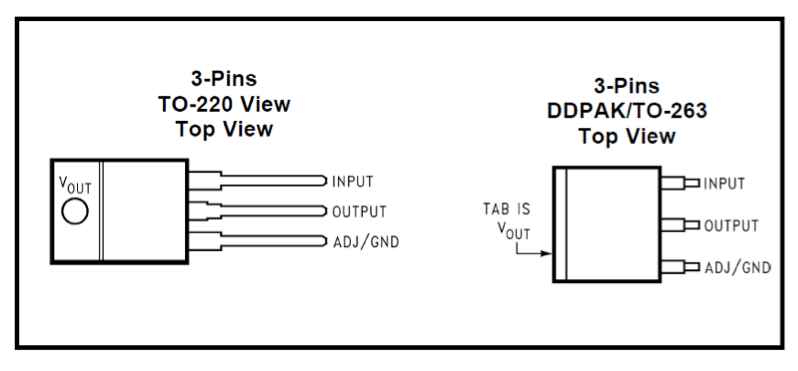# Digital Satellite Finder

By N.S.Sivaramakrishnan

41667

The internal RF Schottky diode peak detector and level shift amplifier converts the RF input signal to a low frequency signal. The detector demonstrates excellent efficiency and linearity over a wide range of input power.

The LTC5508 can be used as a self-standing signal strength measuring receiver and depending on specific application needs, the RSSI output can be split into two branches, providing AC-coupled data output and DC-coupled, RSSI output for signal strength measurements and AGC. The following graph (Figure3) shows that proportionate DC output voltage for the relevant RF input signal. This is coupled to a digital volt meter through R1 & C1.Figure 3. PV characteristics of LTC5508

## Power Supply

A built–in power supply is provided using the two consecutive voltage regulator ICs (LM1086-ADJ) as shown in figure 4. The supply voltages required, are obtained from the satellite receiver itself, using the same RF cable. The inductor 10mH (L1) acts as a RF choke to prevent RF signal from DC biasing to the satellite finder. The voltage regulators are designed using the IC LM1086 to provide 5V & 3.6V respectively. The 5V supply is used for digital display, and 3.6V supply is used for the RF power detector.

The LM1086 adjustable version develops a 1.25V reference voltage, (VREF), between the output terminal (pin2) and the adjust terminal (pin1), at 1.5 A of load current. Two resistors are required to set the output voltage of the adjustable output voltage version of the LM1086. Fixed output voltage versions integrate the adjust resistors. The Load Regulation is 0.1% (Typical) and the Line Regulation is 0.015%. The device component count is very minimal, employing two resistors as part of a voltage divider circuit and an output capacitor for load regulation. The voltage divider for this part is set based on the equations shown below; where R3/R5 is the upper feedback resistor R4/R6 is the lower feedback resistor.

VOUT = 1.25V (1+R6/R5) for 5V output
VOUT = 1.25V (1+R3/R4) for 3.6V outputFigure 4. Power Supply

With a large output capacitor (≥100 μF), and with input instantaneously shorted to ground, the regulator could be damaged. In this case, an external diode (D1) is recommended between the output and input pins to protect the regulator. The capacitors C4 & C6 are used as output filters for both the regulators. The top view pin layout of IC LM1086 is shown below (figure5).Figure 5. Top view of IC1086

## Digital Voltmeter

An accurate digital voltmeter using the IC L7107 from Intersil Corporation, is utilized for digital display. The application circuit is shown in figure6. The IC includes low power, 3.5 digits analog to digital converter, internal circuitry for seven segment decoders, display drivers, reference voltage source and a clock. The power dissipation is less than 10mW and the display stability is very high.

The working of this electronic circuit is very simple. The voltage to be measured is converted into a digital equivalent by the ADC inside the IC and then this digital equivalent is decoded to the seven segment format and then displayed. The ADC used in IC L7107 is dual slope type ADC. The process taking place inside our ADC can be stated as follows. For a fixed period of time the voltage to be measured is integrated to obtain a ramp at the output of the integrator. Then a known reference voltage of opposite polarity is applied to the input of the integrator and allowed to ramp until the output of integrator becomes zero.

### Input voltage comparison & conversion

The time taken for the negative slope to reach zero is measured in terms of the IC’s clock cycle and it will be proportional to the voltage under measurement. In simple words, the input voltage is compared to an internal reference voltage and the result is converted in a digital format. The 14.2 mm (0.56 inch) LED seven segment displays are connected are available as common anode (DISP1 – DISP4). The resistor R13 and C13 are used to set the frequency of IC’s internal clock. Capacitor C12 neutralizes the fluctuations in the internal reference voltage and increases the stability of the display. A 0.1μF capacitor gives good results in most of the applications.

#### 1 COMMENT

1.adel

good